# Random Walk on Lattice

Following the spirit of the previous lecture, we start with the random walks. They are very important to study diffusion through the non-homogeneous media, and, of course, polymers. Here we will consider the simple example of the random walk on the square lattice in 2D. This can be a model for diffusion, or for non-self avoiding polymer chain.

We do a random walk, and now we are interested in its trajectory, as this simulates the polymer chain contour. And we are interested in the properties of this trajectory, as they describe the chain conformations, not just the probability density as a function of the coordinates. The important difference now is that we are building the chain, and we cannot stay at the same place, we must move at each step. But the direction where we move is decided by the MC procedure.

We consider an example of a polar chain with each bond having a dipole momentof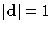, parallel to the bond direction, in the uniform electric field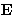. This model can actually describe polymer chain in the flux, and liquid-crystalline ordering. The chain is not self-avoiding, and all segments are independent. Each segment contributes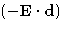to the energy. Probability distribution for conformation of a N-segment chain, described by the set of bond vectors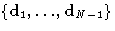is: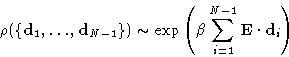To reproduce this we have to choose the direction of each bond vectorwith the weight, proportional to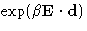, and because we are on a square lattice, we choose one of four possibilities. The probability for each of the choices is (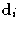is the bond vector for the i-th direction):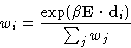MC Algorithm
1.
Choose position of the first segment
2.
Loop to generate a conformation
• Pick the direction of the next bond to grow, based on it's weight wi.
• update the total energy, and the end-to-end vector.
• go back to the beginning of the loop
3.
update current values of the running averages.
4.
go back to step 2

Fortran code Example 3 :
Code.Next: Ising Model Up: Lattice simulations. Random Walk. Previous: Lattice simulations. Random Walk.

© 1997 Boris Veytsman and Michael Kotelyanskii
Thu Nov 6 22:32:26 EST 1997